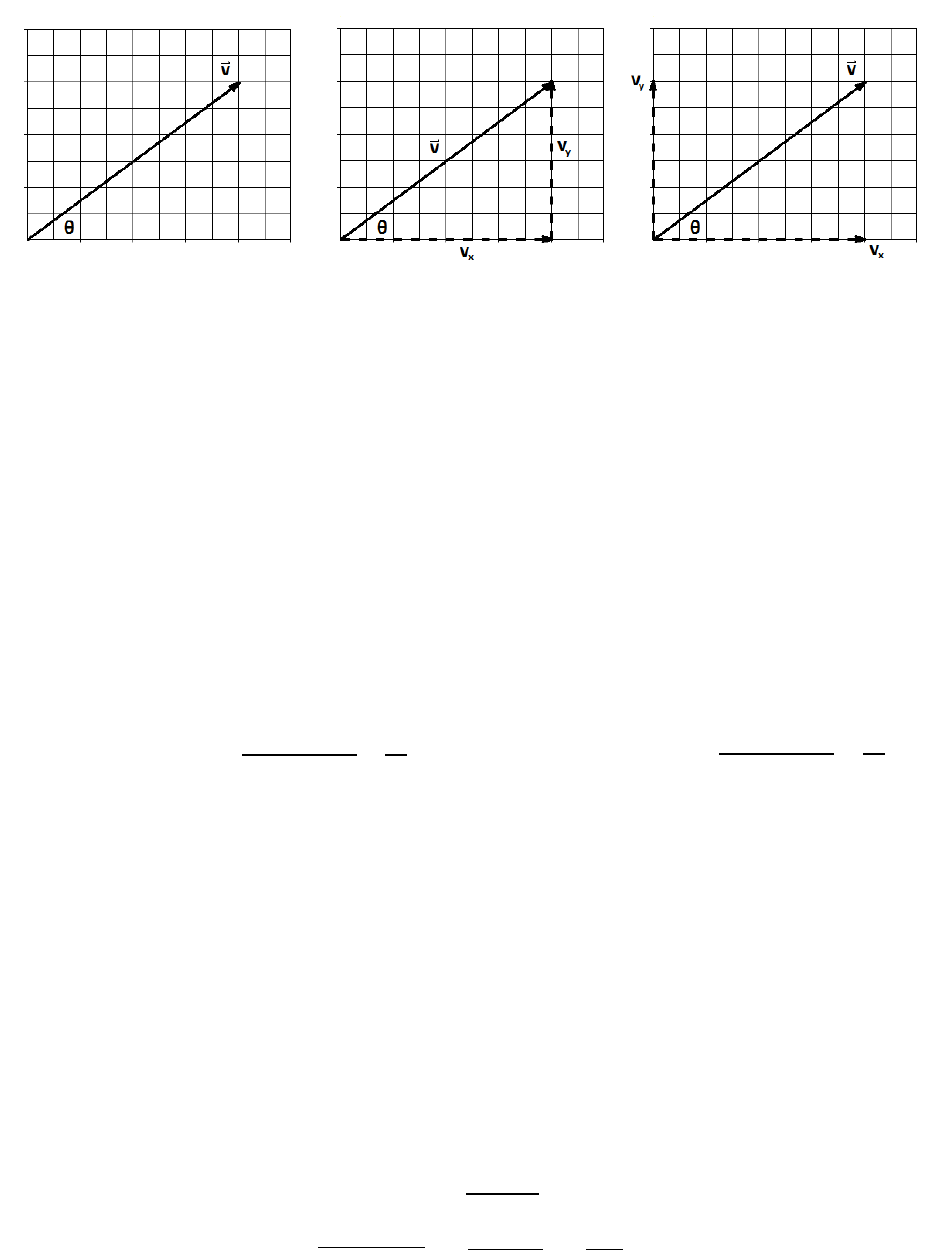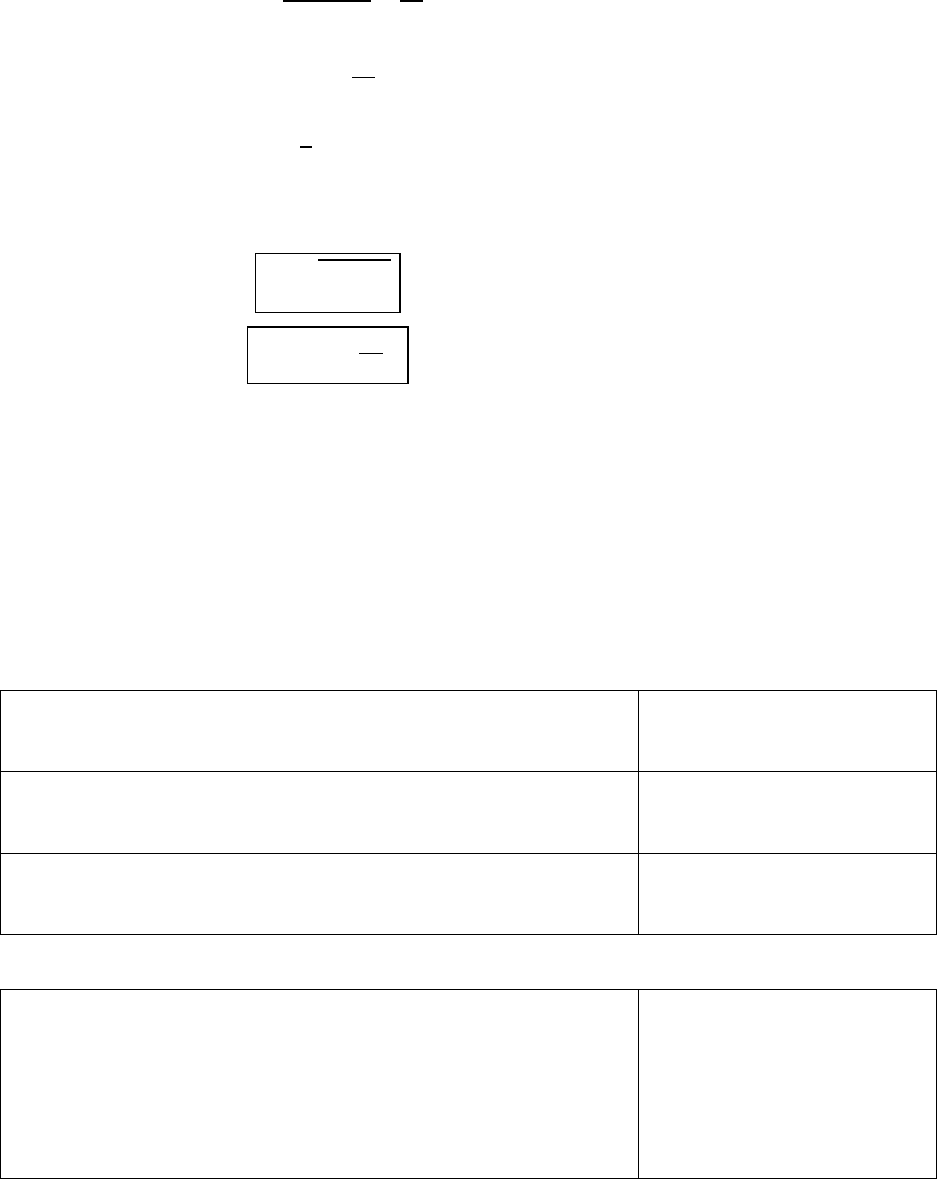# PHYS 2108 Lecture Notes - Lecture 3: Net Force, Hypotenuse, Jeep Wrangler

390 views12 pages
School
Department
Course
Professor3-1
LAB 3:
Vectors
Q: Whee is the estoo i Niholso Hall?
A: About 50 feet from the lab.
This ase is isuffiiet. It’s oe thig to ko that the e’s oo o the oe’s oo is a etai
distance from the lab, but that information is useless without a specified direction (especially in light of
the to oos eig o diffeet floos due to soe oloful ahitetue in the building).
Two categories exist for physical quantities.
Scalars are quantities that are just simply a number. They add and subtract like numbers and
they are probably quantities in which you are familiar. Energy, mass, time and temperature are
all examples of scalar quantities.
Vectors are quantities that have a direction. Velocity and acceleration are vectors, as are forces,
momentum and torque. As you progress further in physics we will find out that fields (electric,
magnetic and gravitational) are also vectors. Vectors do not simply add like numbers and
require very special geometric treatment.
Vectors contain two elements: a magnitude and a direction. The agitude is a sala ad tells us ho
uh stuff the vector is made from, and where the vector is pointed. The directional nature of a vector
is represented in the way we write its symbol: by giving it an arrow as in vector
.
We tend to wrongly interchange some words that are comparable in English, but are distinctly different
i siee like the diffeee etee peisio ad aua. Fo eaple, velocity is not the
same thing as speed. Speed is a number, like 60mph. Velocity is a speed with a direction, like 60mph
South. Speed itself is only the magnitude of the velocity. Additionally, displacement is not the same
thing as distance. Displacement is the net change of location from beginning to end. It has a magnitude
(in this case: a distance) and a direction. Pay attention to the words you use in describing physical
quantities. If the word is a vector, be sure to include its direction when you talk about it in reports and
problem solutions.
Components
A vector is made up of components, that is to sa piees that lie i opletel idepedet dietios.
For something two-dimensional, it has a component in the x-direction and y-direction. In the real world
we also consider a z-component. In a more exotic reality vectors can technically have components in
any number of multiple dimensions, but contemplating such foreign existences can hurt our fragile
minds.
find more resources at oneclass.com
find more resources at oneclass.com
Unlock document

This preview shows pages 1-3 of the document.
Unlock all 12 pages and 3 million more documents.3-2
Consider a vector
that is 10 units long and oriented at 36.8699° above the x-axis (left figure). In
thinking about the components, make the vector the hypotenuse of an imaginary right triangle and put
the x- and y-components (vx and vy) on the sides of the triangle (middle figure). Finally, the length of the
y-component is the same whether we leave it on the edge of the triangle or slide it back to the origin
ight figue. The stadad epesetatio of a eto’s opoets is the laout i the ightost
figure, but until you are comfortable working with them you may find the middle figure most useful in
understanding the mathematics.
Copoets ae kid of a set of tu-by-tu dietios fo ho to eah the ed of a eto. The -
and y-components of this 10 unit-long vector are obvious because this is a special triangle with perfect
sides, and because we can count the squares. The components are 8 units to the right, and 6 up.
Typical etos ae’t so eas ad ust e oke ito opoets tigooetially. In understanding
trig functions, some definitions about the anatomy of a triangle are required. In the middle figure, the x-
component, vx, lies right next to the agle θ ad is alled the adjacent side. The component vy lies
across the tiagle fo the agle θ and is called the opposite side. Let’s t a fe tigooet
formulas and see what happens:
 



As ou gai oe epeiee, ou’ll e ale to eogize hih opoet is which and skip directly to
the equations for vx and vy just from looking at the sketch. Until then, draw out the triangle and start
ith a guess of si θ ad os θ ith opposites ad adjaets to see hih oespods to hih. The x
component will not always e a os θ, ad ie esa fo y. It will depend on which angle is labeled in
the tiagle if e had hose to easue θ fo the -axis instead of the x-axis, they would flip).
We can work in reverse order and find the magnitude and angle if we know the components. If the vx
and vy opoets ae legs i a tiagle, e a use Pthagoea’s Theoe to fid the hpoteuse ad
use some trig functions to discover the angle.
  
find more resources at oneclass.com
find more resources at oneclass.com
Unlock document

This preview shows pages 1-3 of the document.
Unlock all 12 pages and 3 million more documents.3-3
Usig the figue o the peious page to idetif the loatio of θ, e a hoose opoets that ae
 


Use the definition of a tangent.
Take the inverse tangent to
isolate the agle θ.


Technically, you can use sines or cosines to find the angle also, but they each use the hypotenuse. If you
already know the components, it’s uh oe diet to sipl use the taget. I sua:
Eqn. (3-1)
Magnitude of a Vector

Eqn. (3-2)
Orientation of a Vector
(if measured from x-axis)
CAUTION: A calculator is a fairly unsophisticated device that can be confused by directions.
For example, if both the x- and y-components are negative, the calculator will
give you a small positive value for the angle. Always draw a picture of your two
components and the resultant vector in order to identify exactly where the
agle θ lies, and clearly describe it in the vector.
Representing a Vector
There are several ways to represent a vector in a way that expresses both its magnitude and direction.
For the 10-uit eto e’e ee okig ith, it a e epeseted in Magnitude and Direction
Notation as:
10 @ + 36.8699°
All angles measured starting
from the +x-axis and may be
anything from 0 - 360°
10 @ 36.8699° above the +x-axis
Specify the angle as measured
from any + or axis.
10 @ 53.1301° to the right of the +y-axis
10 @ 36.8699° North of East
Treat the axes like a compass
rose and specify your
reference.
10 @ 53.1301° East of North
Another system involves representing vectors by their components, Unit Vector Notation.
o
Each component is explicitly
stated and the direction is
indicated by i-hat, j-hat or k-
hat. Some books use x,y,z
hats, othes use i,j,k hats.
A shorthand is to simply use
angled brackets.
o
< 8, 6 > or < 8, 6, 0 >
find more resources at oneclass.com
find more resources at oneclass.com
Unlock document

This preview shows pages 1-3 of the document.
Unlock all 12 pages and 3 million more documents.

## Document Summary

Vectors (cid:862)whe(cid:396)e is the (cid:396)est(cid:396)oo(cid:373) i(cid:374) ni(cid:272)holso(cid:374) hall? (cid:863) Scalars are quantities that are just simply a number. They add and subtract like numbers and they are probably quantities in which you are familiar. Energy, mass, time and temperature are all examples of scalar quantities. Velocity and acceleration are vectors, as are forces, momentum and torque. As you progress further in physics we will find out that fields (electric, magnetic and gravitational) are also vectors. Vectors do not simply add like numbers and require very special geometric treatment. Vectors contain two elements: a magnitude and a direction. The (cid:373)ag(cid:374)itude is a s(cid:272)ala(cid:396) a(cid:374)d tells us (cid:862)ho(cid:449) (cid:373)u(cid:272)h stuff(cid:863) the vector is made from, and where the vector is pointed. The directional nature of a vector is represented in the way we write its symbol: by giving it an arrow as in vector .

## Get access

\$8 USD/m\$10 USD/m
Billed \$96 USD annuallyHomework Help
Study Guides
Textbook Solutions
Class Notes
Textbook Notes
Booster Class
Class+
\$8 USD/m
Billed \$96 USD annuallyHomework Help
Study Guides
Textbook Solutions
Class Notes
Textbook Notes
Booster Class